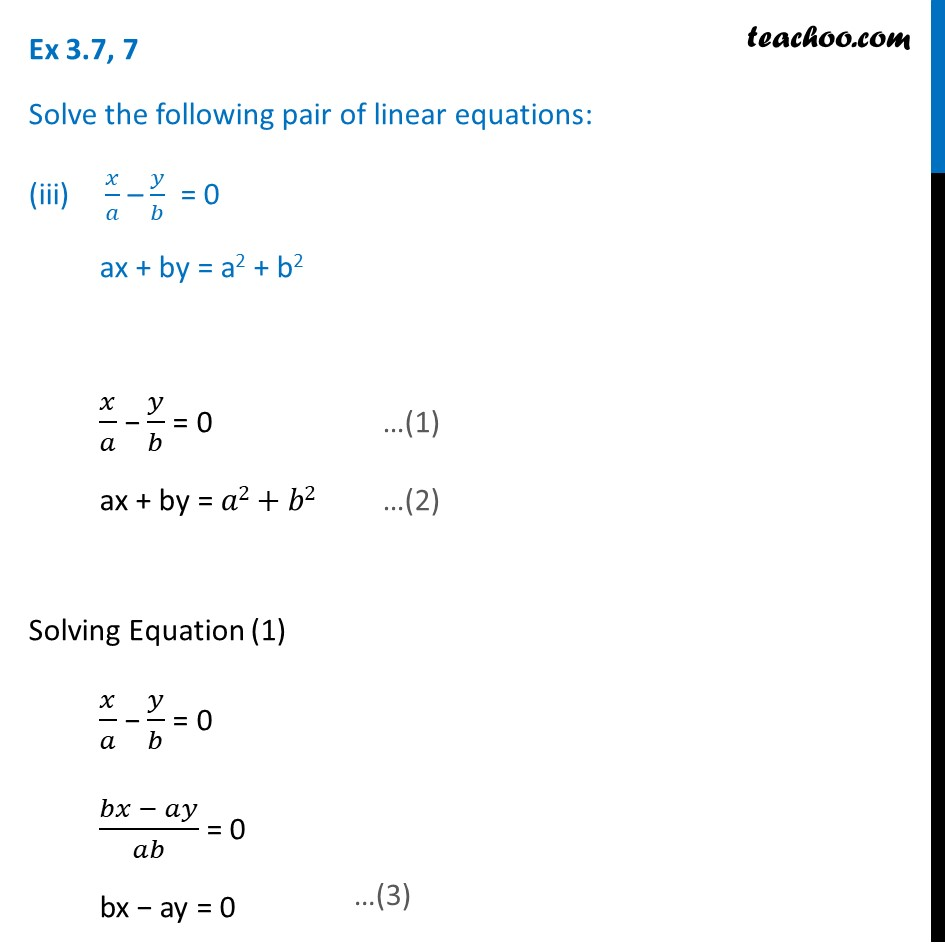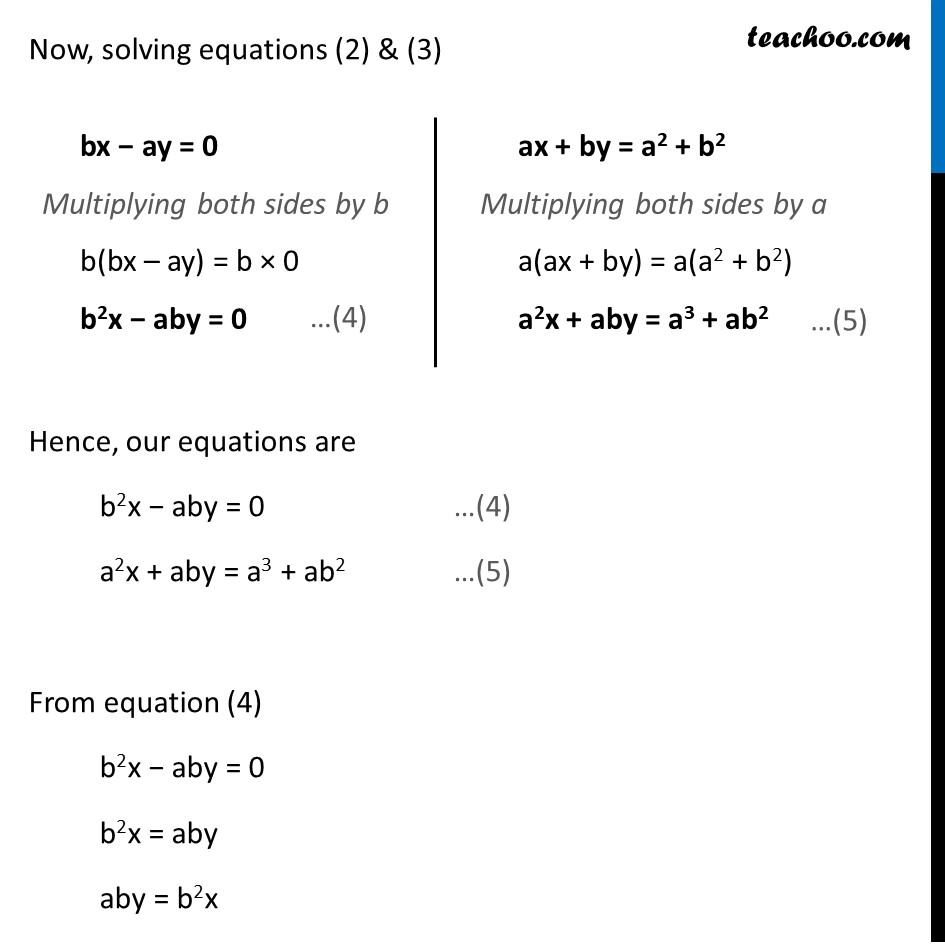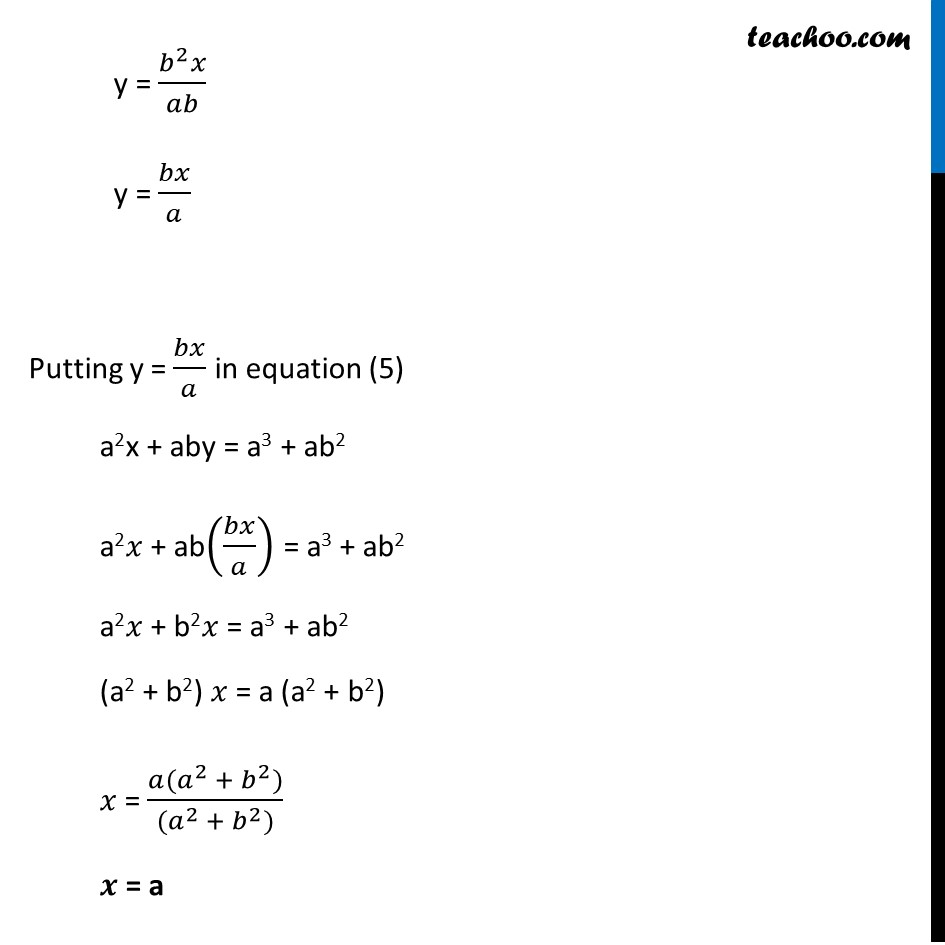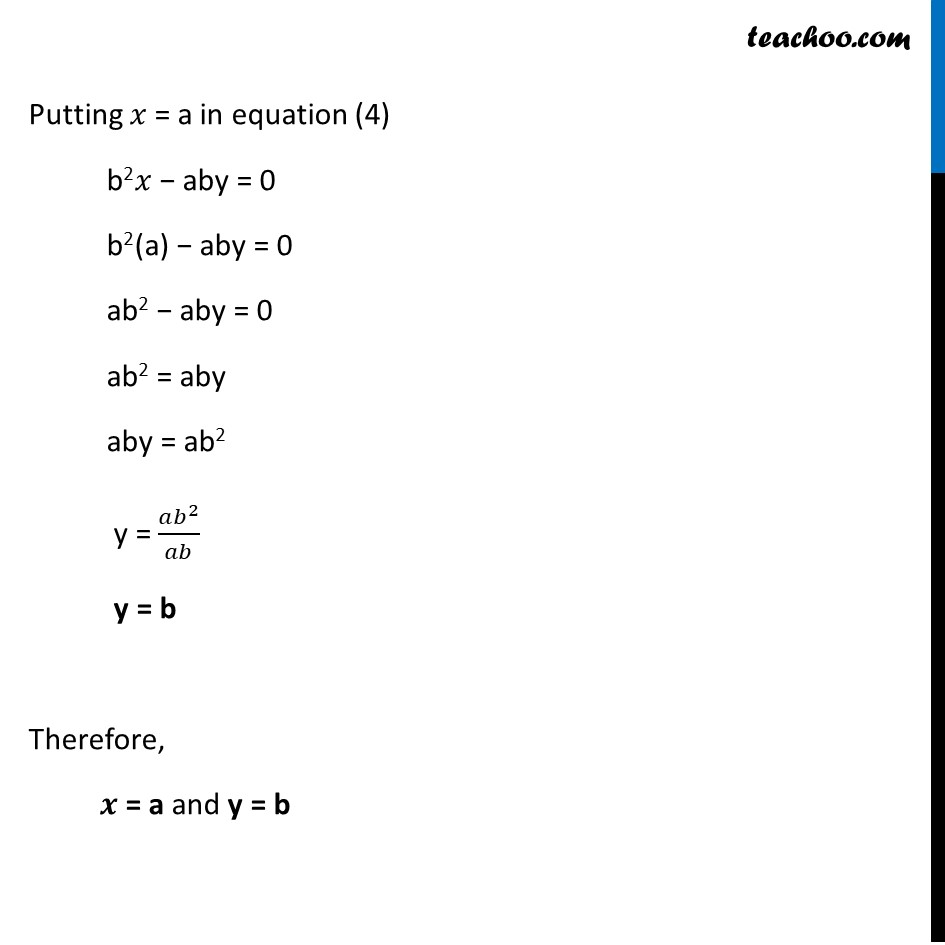1. Chapter 3 Class 10 Pair of Linear Equations in Two Variables (Term 1)
2. Serial order wise
3. Ex.3.7 (Optional)

Transcript

Ex 3.7, 7 Solve the following pair of linear equations: (iii) 𝑥/𝑎 – 𝑦/𝑏 = 0 ax + by = a2 + b2 𝑥/𝑎 − 𝑦/𝑏 = 0 …(1) ax + by = 𝑎2+𝑏2 …(2) Solving Equation (1) 𝑥/𝑎 − 𝑦/𝑏 = 0 (𝑏𝑥 − 𝑎𝑦)/𝑎𝑏 = 0 bx − ay = 0 Now, solving equations (2) & (3) bx − ay = 0 Multiplying both sides by b b(bx – ay) = b × 0 b2x − aby = 0 ax + by = a2 + b2 Multiplying both sides by a a(ax + by) = a(a2 + b2) a2x + aby = a3 + ab2 Hence, our equations are b2x − aby = 0 …(4) a2x + aby = a3 + ab2 …(5) From equation (4) b2x − aby = 0 b2x = aby aby = b2x y = (𝑏^2 𝑥)/𝑎𝑏 y = 𝑏𝑥/𝑎 Putting y = 𝑏𝑥/𝑎 in equation (5) a2x + aby = a3 + ab2 a2𝑥 + ab(𝑏𝑥/𝑎) = a3 + ab2 a2𝑥 + b2𝑥 = a3 + ab2 (a2 + b2) 𝑥 = a (a2 + b2) 𝑥 = (𝑎(𝑎^2 + 𝑏^2))/((𝑎^2 + 𝑏^2)) 𝒙 = a Putting 𝑥 = a in equation (4) b2𝑥 − aby = 0 b2(a) − aby = 0 ab2 − aby = 0 ab2 = aby aby = ab2 y = (𝑎𝑏^2)/𝑎𝑏 y = b Therefore, 𝒙 = a and y = b